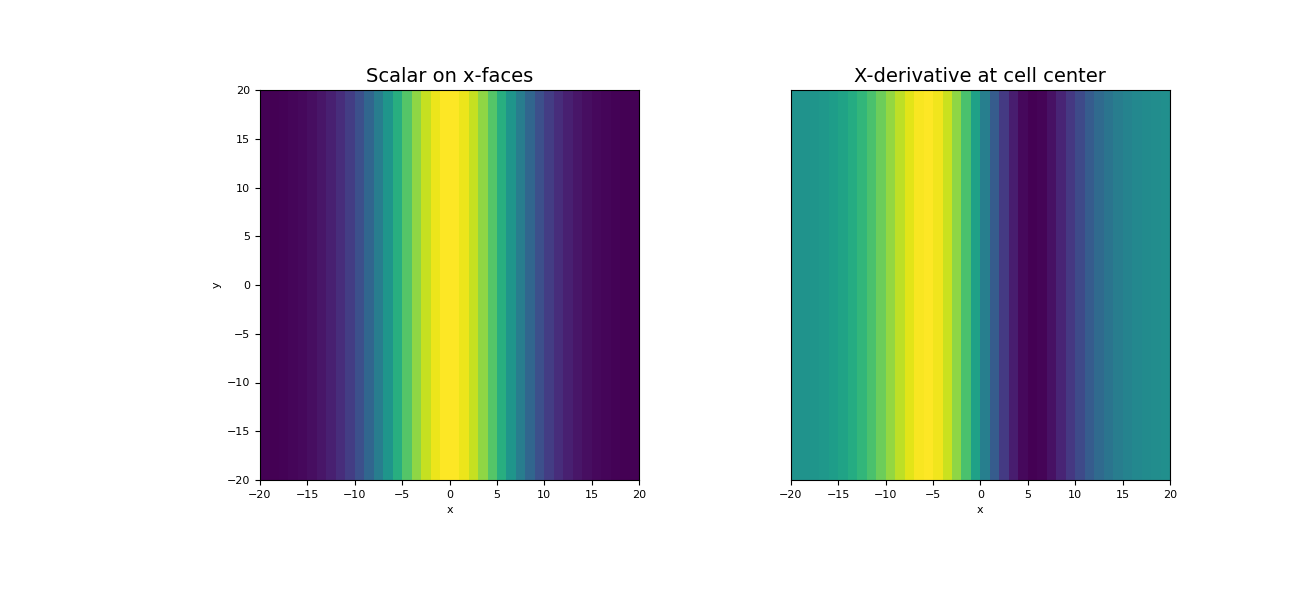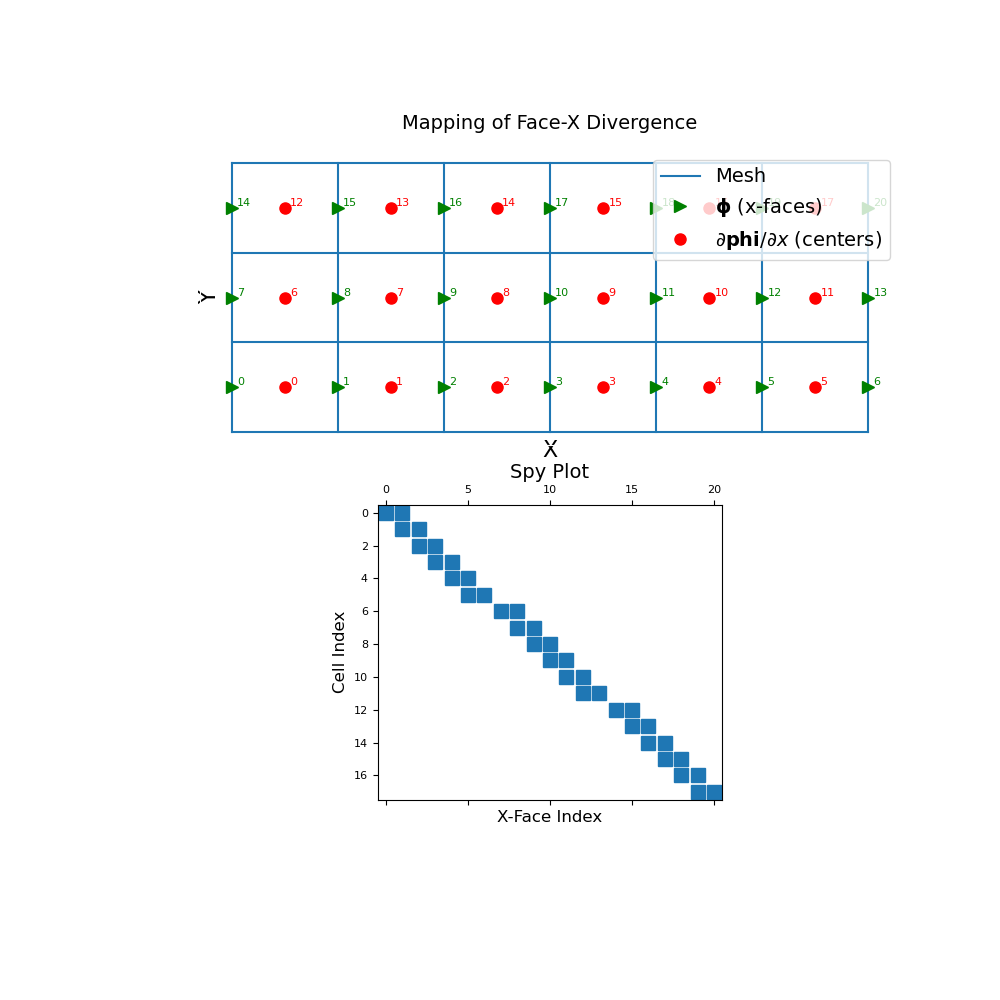# discretize.CylindricalMesh.face_x_divergence¶

property CylindricalMesh.face_x_divergence

X-derivative operator (x-faces to cell-centres)

This property constructs a 2nd order x-derivative operator which maps from x-faces to cell centers. The operator is a sparse matrix $$\mathbf{D_x}$$ that can be applied as a matrix-vector product to a discrete scalar quantity $$\boldsymbol{\phi}$$ that lives on x-faces; i.e.:

dphi_dx = Dx @ phi


For a discrete vector whose x-component lives on x-faces, this operator can also be used to compute the contribution of the x-component toward the divergence.

Returns
(n_cells, n_faces_x) scipy.sparse.csr_matrix

The numerical x-derivative operator from x-faces to cell centers

Examples

Below, we demonstrate 1) how to apply the face-x divergence operator, and 2) the mapping of the face-x divergence operator and its sparsity. Our example is carried out on a 2D mesh but it can be done equivalently for a 3D mesh.

We start by importing the necessary packages and modules.

>>> from discretize import TensorMesh
>>> import numpy as np
>>> import matplotlib.pyplot as plt
>>> import matplotlib as mpl


For a discrete scalar quantity $$\boldsymbol{\phi}$$ defined on the x-faces, we take the x-derivative by constructing the face-x divergence operator and multiplying as a matrix-vector product.

>>> h = np.ones(40)
>>> mesh = TensorMesh([h, h], "CC")


Create a discrete quantity on x-faces

>>> faces_x = mesh.faces_x
>>> phi = np.exp(-(faces_x[:, 0] ** 2) / 8** 2)


Construct the x-divergence operator and apply to vector

>>> Dx = mesh.face_x_divergence
>>> dphi_dx = Dx @ phi


Plot the original function and the x-divergence

Expand to show scripting for plot
>>> fig = plt.figure(figsize=(13, 6))
>>> w = np.r_[phi, np.ones(mesh.nFy)]  # Need vector on all faces for image plot
>>> mesh.plot_image(w, ax=ax1, v_type="Fx")
>>> ax1.set_title("Scalar on x-faces", fontsize=14)
>>> mesh.plot_image(dphi_dx, ax=ax2)
>>> ax2.set_yticks([])
>>> ax2.set_ylabel("")
>>> ax2.set_title("X-derivative at cell center", fontsize=14)
>>> plt.show()The discrete x-face divergence operator is a sparse matrix that maps from x-faces to cell centers. To demonstrate this, we construct a small 2D mesh. We then show the ordering of the elements in the original discrete quantity $$\boldsymbol{\phi}}$$ and its x-derivative $$\partial \boldsymbol{\phi}}/ \partial x$$ as well as a spy plot.

Expand to show scripting for plot
>>> mesh = TensorMesh([[(1, 6)], [(1, 3)]])
>>> fig = plt.figure(figsize=(10, 10))
>>> mesh.plot_grid(ax=ax1)
>>> ax1.plot(
...     mesh.faces_x[:, 0], mesh.faces_x[:, 1], "g>", markersize=8
... )
>>> for ii, loc in zip(range(mesh.nFx), mesh.faces_x):
...     ax1.text(loc+0.05, loc+0.02, "{0:d}".format(ii), color="g")
>>> ax1.plot(
...     mesh.cell_centers[:, 0], mesh.cell_centers[:, 1], "ro", markersize=8
... )
>>> for ii, loc in zip(range(mesh.nC), mesh.cell_centers):
...     ax1.text(loc+0.05, loc+0.02, "{0:d}".format(ii), color="r")

>>> ax1.set_xticks([])
>>> ax1.set_yticks([])
>>> ax1.spines['bottom'].set_color('white')
>>> ax1.spines['top'].set_color('white')
>>> ax1.spines['left'].set_color('white')
>>> ax1.spines['right'].set_color('white')
>>> ax1.set_title("Mapping of Face-X Divergence", fontsize=14, pad=15)
>>> ax1.legend(
...     ['Mesh', '$\mathbf{\phi}$ (x-faces)', '$\partial \mathbf{phi}/\partial x$ (centers)'],
...     loc='upper right', fontsize=14
... )